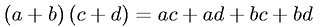Equations > Algebra > Polynomials > Polynomial FOIL operation

Polynomial FOIL operationLatex Code:

MathML Code:

 $\left(a+b\right)\left(c+d\right)=\mathrm{ac}+\mathrm{ad}+\mathrm{bc}+\mathrm{bd}$

MathType 5.0: